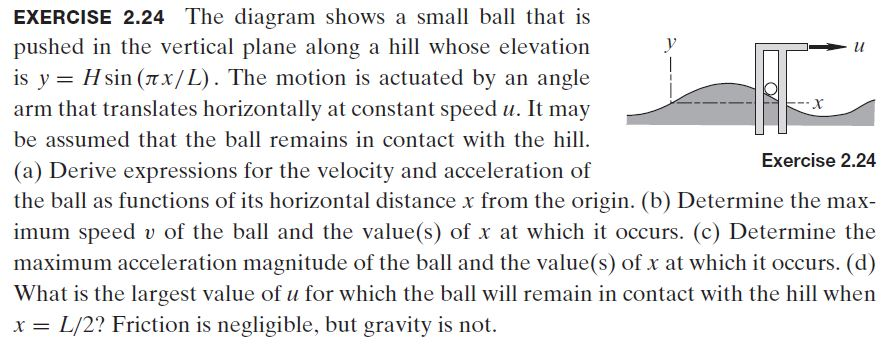Home / Answered Questions / Other / exercise-2-24-the-diagram-shows-a-small-ball-that-is-pushed-in-the-vertical-plane-along-a-hill-whose-aw376

# (Solved): EXERCISE 2.24 The Diagram Shows A Small Ball That Is Pushed In The Vertical Plane Along A Hill Whose...EXERCISE 2.24 The diagram shows a small ball that is pushed in the vertical plane along a hill whose elevation is y = H sin (ax/L). The motion is actuated by an angle arm that translates horizontally at constant speed u. It may be assumed that the ball remains in contact with the hill. (a) Derive expressions for the velocity and acceleration of Exercise 2.24 the ball as functions of its horizontal distance x from the origin. (b) Determine the max- imum speed v of the ball and the value(s) of x at which it occurs. (C) Determine the maximum acceleration magnitude of the ball and the value(s) of x at which it occurs. (d) What is the largest value of u for which the ball will remain in contact with the hill when x = L/2? Friction is negligible, but gravity is not.

We have an Answer from Expert